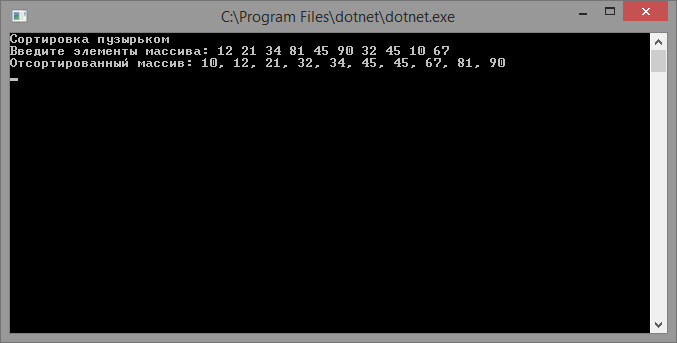# Bubble sort

Updated: 15.09.2019

Bubble sort (bubble sort) is one of the easiest to understand methods for sorting arrays.

## Description of the bubble sort algorithm

The algorithm consists in cyclic passes through the array, for each pass the elements of the array are compared in pairs and, if their order is not correct, then an exchange is carried out. Array traversal is repeated until the array is ordered.

## Implementing bubble sort

``````using System;

class program
{
// elements exchange method
static void Swap(ref int e1, ref int e2)
{
var temp = e1;
e1 = e2;
e2 = temp;
}

// sort by bubble
static int[] BubbleSort(int[] array)
{
var len = array.Length;
for (var i = 1; i < len; i++)
{
for (var j = 0; j < len - i; j++)
{
if (array[j] > array[j + 1])
{
Swap(ref array[j], ref array[j + 1]);
}
}
}

return array;
}

static void Main(string[] args)
{
Console.WriteLine("Bubble Sort");
Console.Write("Enter the elements of the array:");
var parts = Console.ReadLine().Split(new[] { "", ",", ";" }, StringSplitOptions.RemoveEmptyEntries);
var array = new int[parts.Length];
for (int i = 0; i < parts.Length; i++)
{
array[i] = Convert.ToInt32(parts[i]);
}

Console.WriteLine("Sorted array: {0}", string.Join(",", BubbleSort(array)));

The result of the program: